# Lesson 6: Distribution of Sample Means & The Central Limit Theorem

Jump to: navigation, search

These optional videos discuss the contents of this lesson.

## 1 Lesson Outcomes

By the end of this lesson, you should be able to:

• Explain how a sampling distribution is created.
• Determine the mean, standard deviation and shape of a distribution of sample means.
• State and apply the Central Limit Theorem and the Law of Large numbers.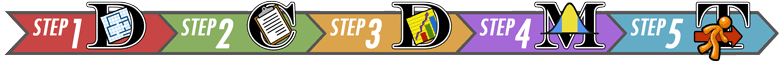## 2 Distribution of Sample Means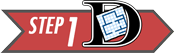Step 1: Design the Study

Mosquitoes and other biting insects carry malaria. In an attempt to curb the spread of malaria and save lives, the pesticide DDT was used for many years to control the insect population—even indoors. Unfortunately, this pesticide does not break down quickly in nature and is very harmful to humans.

A metabolite of DDT (called DDE) is also very dangerous. A metabolite is the byproduct that occurs when our body breaks down a substance. When DDT is broken down in humans, DDE is one of the metabolites that remains from the original DDT.

Scientists have shown that DDT and its metabolites cause reproductive problems in humans and other animals. When a pregnant woman has a contamination level as low as 10 mg/kg of DDE in her body, she is much more likely to give birth to an underweight baby or to deliver prematurely (Wells & Leonard, 2006).

A study of 45 pregnant women who have been exposed to DDT / DDE was conducted using a convenience sample (Bornman, MS. 2005). Global Solutions Unlimited (GSU) will analyze the data from this study. These data will be used to assess the prevalence of DDT in drinking water.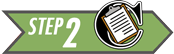Step 2: Collect Data

After selecting specific women to be sampled, researchers measured the level of DDE contamination for each of these randomly selected women.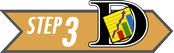Step 3: Describe the Data

The researchers computed the mean and standard deviation of the observed levels of exposure for the 45 women. They found the mean observed contamination level was 24.75 mg/kg, and the highest level of contamination was 419.91 mg/kg! (Bornman, MS. 2005)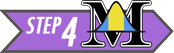Step 4: Make Inferences

The researchers only obtained one sample mean, $\bar x$. They do not get to see any other data. They only had enough time, funding, and other resources to survey the 45 women.

If the researchers had selected a different random sample of pregnant women, the mean contamination of the women in the sample would undoubtedly be different. However, this did not happen. The researchers do not get to see the contamination levels for any other women. They only collected this one sample.

This is how research is often conducted...repeated samples are not typically drawn, so we do not see the distribution of sample means.

Researchers typically see only one value of the sample mean, $\bar x$. Unlike the simulations you have experienced in this lesson, in the real world, you only get to see the results of one experiment: yours.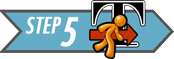Step 5: Take Action

In this study, there was a lot of evidence that the mean DDE contamination in the pregnant women was greater than 10 mg/kg. The $P$-value for the test to determine if the DDE contamination in the women’s bodies exceeds 10 mg/kg is approximately 0.02. There is reason for concern. There is sufficient evidence to suggest that the levels of DDE contamination are too high among South African women.

### 2.1 Introduction to Sampling Distributions

Please do the following:

• Step 02: Click on the [Begin] button. This will bring up a Java Applet that will demonstrate Sampling Distributions. You will see four graphs. The following paragraphs explain each of these graphs.

The top graph represents the distribution of the population we are studying. This is labeled the “parent population.” To the left of this graph is the population mean and standard deviation. We typically don't know the population mean and standard deviation, but for this simulation, we assume they are known!

To the right of the histogram of the parent population is a drop down menu that allows you to choose the type of distribution for your population. We will only look at Normal, Skewed and Uniform.

• Step 03: Before we move to the next step, go to the right of the third graph (labeled "Distribution of Means") and change N=5 to N=25 in the drop down menu. This means that each sample will contain 25 data items from the population.
• Step 04: The graph labeled Sample Data will display the individual data points from a sample. Press the [Animated] button to the right of this graph, and watch a random sample of data items fall from the population. The [Animated] button simulates the collection of a random sample of size n=25 from the parent population. Notice that it calculates the mean and plots the mean of the 25 values in the Distribution of Means graph. (The mean is shown in blue.)
• Step 05: Repeatedly pushing the [Animated] button produces more samples of size 25, calculates the mean of each sample, and then places the mean in the “Distribution of Means” graph. Play with this a few times to make sure you understand the process.
Notice that to the left of the Distribution of Means graph, the mean and standard deviation of the sample means is given. This is the mean and standard deviation of the “Distribution of Means.” In other words, it gives the mean of the means and the standard deviation of the means.
• Step 06: In order to get more data, press the [10,000] button to generate 10,000 samples of size 25 per sample. Soon a histogram of all 10,000 means will be displayed.
We are interested in the shape, center and spread of the “Distribution of Means” graph. This graph represents the distribution of sample means.
• Step 07: Press the [Clear Lower 3] button. This will erase your data and reset the applet. Repeat Steps 04-08. Compare the new graph of the sample means (the third plot) with the graph you originally created. Even though they are based on different simulated draws from the population, they should be very similar.
• Step 08: Press the [Clear Lower 3] button. Then, use the drop down menu below the [Clear Lower 3] button to change the distribution from a normal distribution to a uniform distribution. In a uniform distribution, all the values are equally likely to occur. In this simulation, the applet generates values between 1 and 32. This uniform distribution models rolling a fair, 32-sided die. Repeat steps 04-08 for the uniform distribution. What do you notice about the shape of the distribution of the sample means?
• Step 09: Press the [Clear Lower 3] button. Then, use the drop down menu below the [Clear Lower 3] button to change the distribution from a uniform distribution to a skewed distribution. This represents a right-skewed distribution. Repeat steps 04-08 for the skewed distribution. What do you notice about the shape of the distribution of the sample means?
• Step 10: Press the [Clear Lower 3] button. Then, use the drop down menu below the [Clear Lower 3] button to change the distribution from a skewed distribution to a custom distribution. This allows you to draw any distribution you wish using your mouse. Have fun. Choose a crazy distribution. Repeat steps 04-08 for your custom distribution. Take notice of the shape of the sample means.
• Step 11: Repeat step 10 for different distributions. Be sure to try some that are very skewed, some that are high on the ends and low in the middle, or other crazy shapes. It is important that you press the [Clear Lower 3] button every time you change the distribution. Otherwise the graph of the means will contain a mixture of the results from the different distributions.

### 2.2 What is the Distribution of Sample Means?

The sampling distribution of the sample mean is the set of all possible values of $\bar x$ that could occur. You have seen several examples of sampling distributions as you have plotted many means in the simulations and observed the approximately normal distribution that occurs. In the real world, you only observe your sample mean. You do not get to view the distribution. However, the fact that you sample randomly means that you could easily have drawn a different sample and had a different sample mean, $\bar x$. There are many possible sample means; you can think about your $\bar x$ as a sample of size 1 from the distribution of all the possible sample means that could have occurred.

## 3 The Central Limit Theorem

In the study of DDE levels in the South African women, we never saw the distribution of sample means. We only observed one sample mean, $\bar x$. Even though we do not get to see the distribution of all possible sample means, since the sample size ($n = 45$) was large, we can be assured that the sample mean $\bar x$ can be considered as one drawn from a normally distributed population of possible sample means.

You have observed in the simulations that if the sample size is large, the random variable $\bar x$ will be approximately normally distributed. In other words, the sampling distribution of the sample mean will be approximately normal if the sample size is sufficiently large. This important result is called the Central Limit Theorem. This is arguably the most important concept in all of Statistics.

The Central Limit Theorem works for every population where the standard deviation is defined (is not infinite). In other words, it will work for any distribution you find in the real world.

### 3.1 When is $\bar x$ Normal?

How many observations are required so that the Central Limit Theorem will assure that the distribution of sample means will be approximately normal? The answer is, “it depends.”

• If the parent population is normal, then the sampling distribution of $\bar x$ will always be normally distributed, no matter how many observations are selected.
• If the parent population is not normal, then the sampling distribution $\bar x$ will be approximately normally distributed, if the sample size is large enough.
• If the parent population is almost normal (e.g. mound-shaped and nearly symmetrical), then a sample of size n = 5 will probably be sufficient to assure that $\bar x$ will be approximately normally distributed.
• If the parent population is heavily skewed, then it will require a larger sample size to be assured that $\bar x$ will be normally distributed. For most moderately skewed distributions, a sample size of around 30 is traditionally thought to be sufficiently large to assure that $\bar x$ will be approximately normally distributed. This is not a definitive number, but is a rule of thumb.
• For tremendously skewed distributions (e.g., the distribution of lottery payouts), a much larger sample will be required before the distribution of sample means is approximately normal. This may require billions of observations. Simulation can be used to determine if a particular sample size is sufficient. For this course, if the sample size is at least 30, we will conclude that the sampling distribution of $\bar x$ will be approximately normal.
Answer the following question:
1. There are two ways that $\bar x$ can be (approximately) normally distributed. What are they?
• $\bar x$ will be normally distributed if the data were drawn from a normal population.
• $\bar x$ will be approximately normally distributed if the sample size is sufficiently large.

## 4 Mean and Standard Deviation of the Distribution of Sample Means

The following facts are always true. They do not depend on the Central Limit Theorem. They do not depend on the sample size. These facts hold for the sample mean $\bar x$ of any simple random sample of size $n$ drawn from a population with mean $\mu$ and standard deviation $\sigma$.

• The mean of the sample means is $\mu$
• The standard deviation of the sample means is $\sigma / \sqrt{n}$

Remember, these facts are always true. They do not depend on normality or the sample size. So, even though these facts are often used in conjunction with the Central Limit Theorem, they do not depend on it.

Think about the simulations you have observed. The mean of the sample means was always aligned with $\mu$. Also, if you took samples larger than $n = 1$, the standard deviation of sample means was always smaller than $\sigma$.

Answer the following questions:
2. The amount of time passengers spend waiting for a bus on a particular urban route follows a distribution that has a mean of 8.7 minutes with a standard deviation of 2.2 minutes. Transportation officials observed the waiting times for a random sample of $n=121$ individual passengers and recorded the sample mean, $\bar x$. We can think of this sample mean as one value observed out of all the possible sample means that could have been observed. What is the mean of the distribution of all possible sample means?

$\mu = 8.7~\text{minutes}$

3. Use the information in the previous problem to answer this question: What is the standard deviation of the distribution of all possible sample means?

$\frac{\sigma}{\sqrt{n}} = \frac{2.2}{\sqrt{121}} = 0.2~\text{minutes}$

## 5 The Law of Large Numbers

Answer the following questions:
4. We just learned that the standard deviation of sample means is $\displaystyle{ \sigma \over \sqrt{n} }$. What happens to the standard deviation of sample means when the sample size is increased?
If the sample size, $n$, is increased, then the standard deviation of the sample mean will decrease. The fraction will get smaller.

5. If the standard deviation of the sample mean gets smaller, what happens to the values of $\bar x$?
The values that will be observed will be very close to each other and therefore close to $\mu$, if the sample size is large.

The result you have discovered in the previous two questions is called the Law of Large Numbers. The Law of Large Numbers states that if the sample size is large, then the sample mean will typically be close to the population mean, $\mu$. This happens because the standard deviation $\sigma / \sqrt{n}$ will get smaller.

Notice that this is very different from the Central Limit Theorem. The Central Limit Theorem states that if the sample size is large that $\bar x$ will be approximately normal. The Law of Large numbers states that the sample mean $\bar x$ will be close to $\mu$.

Take a moment to study the difference between the Central Limit Theorem and the Law of Large Numbers. They are very different, but students tend to confuse them.

## 6 Review of Key Concepts

In order to "do statistics" we need to compute probabilities. This requires we know the distribution of the population of interest. The most important distributions in this course are the normal distributions, which were introduced in Lesson 05. In this lesson, we are interested in another very important distribution: the distribution of all the sample means, $\bar{x}$. In order to do statistics with $\bar{x}$ we need the distribution of $\bar{x}$ to be normal. There are two situations when the distribution of $\bar{x}$ is guaranteed to be normal (or as near as makes no difference). They are:

• If the parent population is normal, the distribution of the sample means $\bar{x}$ will be normal, for every sample size $n$.
• Even if the parent population is not normal, the Central Limit Theorem guarantees that the distribution of the sample mean $\bar{x}$ will be approximately normal if the sample size $n$ is large enough. For this course, if $n \geq 30$, we will say the distribution of the sample means will be approximately normal (even if the parent population is not normal).

The mean and standard deviation of $\bar{x}$ are:

• The mean $\mu_{\bar{x}}$ of the sample means is the population mean $\mu$.
• The standard deviation $\sigma_{\bar{x}}$ of the sample means is the population standard deviation $\sigma$ divided by the square root of $n$, $\sigma / \sqrt{n}$

## 7 Summary

Remember...
• The distribution of sample means is a distribution of all possible sample means ($\bar x$) for a particular sample size. It has a mean of $\mu$ and a standard deviation of $\sigma/\sqrt{n}$.
• The distribution of sample means is normal when $\bar x$ is normally distributed or when, thanks to the Central Limit Theorom (CLT), our sample size ($n$) is large.
• The Law of Large Numbers states that as the sample size ($n$) gets larger, the sample mean ($\bar x$) will get closer to the population mean ($\mu$).

## 8 Navigation

 Previous Reading: Lesson 05: Normal Distributions This Reading: Lesson 06: Distribution of Sample Means; Central Limit Theorem Next Reading: Lesson 07: Probability Calculations involving a Mean Response